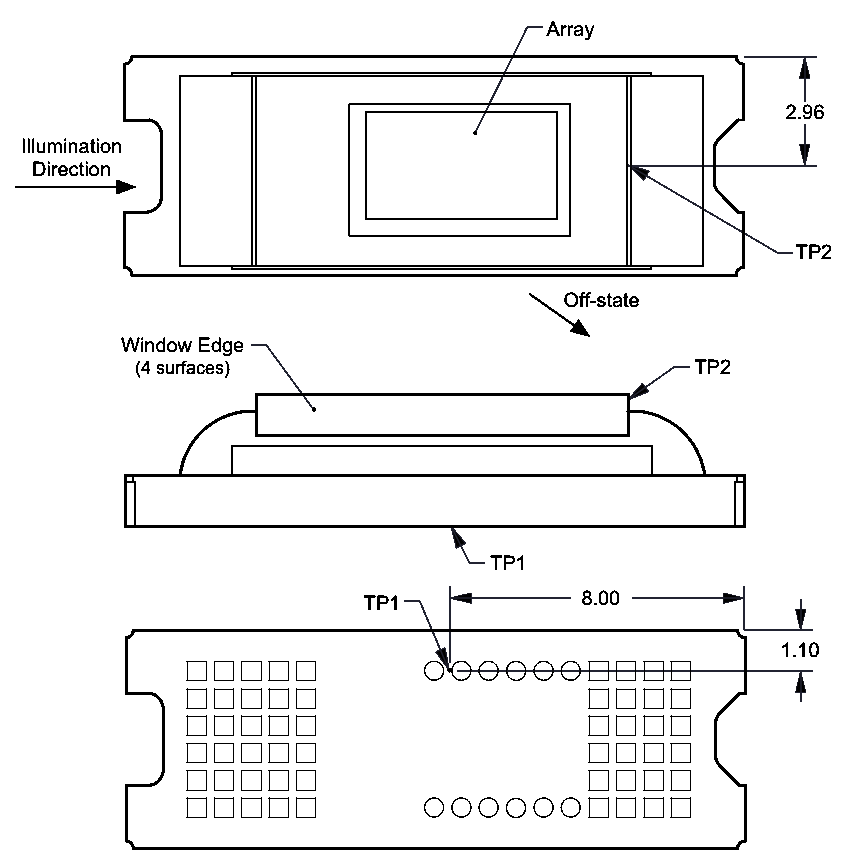ZHCSIP9D july   2018  – july 2023

PRODUCTION DATA

1.   1
2. 特性
3. 显示应用
4. 说明
5. Revision History
6. Pin Configuration and Functions
7. Specifications
8. Detailed Description
9. Application and Implementation
1. 8.1 Application Information
2. 8.2 Typical Application
10. Power Supply Recommendations
11. 10Layout
12. 11Device and Documentation Support
1. 11.1 Device Support
2. 11.2 Chipset Resources
3. 11.3 接收文档更新通知
4. 11.4 支持资源
6. 11.6 静电放电警告
7. 11.7 术语表
13. 12Mechanical, Packaging, and Orderable Information

• FQP|54

## 7.6 Micromirror Array Temperature CalculationFigure 7-1 DMD Thermal Test Points

Micromirror array temperature cannot be measured directly, therefore it must be computed analytically from measurement points on the outside of the package, the package thermal resistance, the electrical power, and the illumination heat load. The relationship between array temperature and the reference ceramic temperature (thermal test TP1 in Figure 7-1) is provided by the following equations:

TARRAY = TCERAMIC + (QARRAY × RARRAY-TO-CERAMIC)
QARRAY = QELECTRICAL + QILLUMINATION

where

• TARRAY = Computed array temperature (°C)
• TCERAMIC = Measured ceramic temperature (°C) (TP1 location)
• RARRAY-TO-CERAMIC = Thermal resistance of package specified in Section 6.5 from array to ceramic TP1 (°C/Watt)
• QARRAY = Total DMD power on the array (W) (electrical + absorbed)
• QELECTRICAL = Nominal electrical power (W)
• QINCIDENT = Incident illumination optical power (W)
• QILLUMINATION = (DMD average thermal absorptivity × QINCIDENT) (W)
• DMD average thermal absorptivity = 0.4

The electrical power dissipation of the DMD is variable and depends on the voltages, data rates and operating frequencies. A nominal electrical power dissipation to use when calculating array temperature is 0.17 Watts. The absorbed power from the illumination source is variable and depends on the operating state of the micromirrors and the intensity of the light source. The equations shown above are valid for a single chip or multichip DMD system. It assumes an illumination distribution of 83.7% on the active array, and 16.3% on the array border.

The sample calculation for a typical projection application is as follows:

QINCIDENT = 2.9 W (measured)
TCERAMIC = 55.0°C (measured)
QELECTRICAL = 0.17 W
QARRAY = 0.17 W + (0.40 × 2.9 W) = 1.33 W
TARRAY = 55.0°C + (1.33 W × 9.0°C/W) = 67.0°C